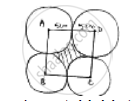Share

# Four Equal Circles, Each of Radius 5 Cm Touch Each Other as Shown in Fig. Find the Area Included Between Them. - CBSE Class 10 - Mathematics

ConceptProblems Based on Areas and Perimeter Or Circumference of Circle, Sector and Segment of a Circle

#### Question

Four equal circles, each of radius 5 cm touch each other as shown in fig. Find the area included etween them.

#### SolutionArea required shaded = (area of square ABCD) – (Area of 4 quadrant)

Side of square = 5cm + 5cm

= 10cm

Area of square = side × side

= 10𝑐𝑚 × 10𝑐𝑚 = 100𝑐𝑚2

Area of quadrant =1/4 (𝑎𝑟𝑒𝑎 𝑜𝑓 𝑐𝑖𝑟𝑐𝑙𝑒 𝑤𝑖𝑡ℎ 𝑟𝑎𝑑𝑖𝑢𝑠 5 𝑐𝑚)

=1/4×pir^2

=1/4×22/7× 5 × 5 = (25 × 3.14) 1/4 cm^2

Area included between circles = (area of square) – 4(area of quadrant)

= 100  – (1/4× 25 × 2.14)

= 100 – 78.5

= 21.5cm2

Is there an error in this question or solution?

#### Video TutorialsVIEW ALL 

Solution Four Equal Circles, Each of Radius 5 Cm Touch Each Other as Shown in Fig. Find the Area Included Between Them. Concept: Problems Based on Areas and Perimeter Or Circumference of Circle, Sector and Segment of a Circle.
S